Thank you for visiting nature.com. You are using a browser version with limited support for CSS. To obtain the best experience, we recommend you use a more up to date browser (or turn off compatibility mode in Internet Explorer). In the meantime, to ensure continued support, we are displaying the site without styles and JavaScript.

Quantum chemistry and charge transport in biomolecules with superconducting circuits

Abstract

We propose an efficient protocol for digital quantum simulation of quantum chemistry problems and enhanced digital-analog quantum simulation of transport phenomena in biomolecules with superconducting circuits. Along these lines, we optimally digitize fermionic models of molecular structure with single-qubit and two-qubit gates, by means of Trotter-Suzuki decomposition and Jordan-Wigner transformation. Furthermore, we address the modelling of system-environment interactions of biomolecules involving bosonic degrees of freedom with a digital-analog approach. Finally, we consider gate-truncated quantum algorithms to allow the study of environmental effects.

Introduction

The field of quantum chemistry arises from the application of quantum mechanics in physical models to explain the properties of chemical and biological systems1,2. The study of complex electronic structures in atoms and molecules encounters the difficulty of the exponential growth of the Hilbert space dimensions with the system size3,4. This fact limits the results reachable with current computers and classical algorithms and strongly suggests to explore the possibilities of new quantum-based tools5,6.

Quantum simulations are a powerful field based on the imitation of the dynamics of a quantum system in a controllable quantum platform7,8. Theoretical and experimental efforts for solving problems in physical chemistry have been performed in technologies such as NMR9, trapped ions10,11,12, photonic systems13,14,15 and superconducting circuits16, among others. Quantum algorithms for the simulation of electronic structures with fermionic degrees of freedom and its optimisation have been widely studied17,18,19,20,21,22,23,24. Environmental effects also play a crucial role in quantum physics, chemistry and biology25,26. Fundamental phenomena such as electronic transport and electron transfer are described through the correlated dynamics of electrons and phonons, involving bosonic and fermionic modes.

Circuit quantum electrodynamics (cQED) is a cutting-edge technology in terms of design versatility, coherent control and scalability27. Indeed, remarkable experimental progress in cQED has enabled the realisation of digital quantum simulations of fermions28, spin systems29 and adiabatic quantum computing30. These aspects, along with the possibility of encoding both fermions and bosons in this platform via digital31,32,33,34 and digital-analog techniques35, make cQED a suitable platform for simulating electronic Hamiltonians36 and dissipative processes.

In this manuscript, we combine efficient digital quantum simulation techniques for electronic Hamiltonians with existing algorithms in quantum chemistry and we analyze the scalability and feasibility according to the state-of-the-art cQED27. In this sense, we study the gate fidelities required for the proposed tasks and the error propagation. We extend these procedures by exploiting the possibility of mimicking bosons in superconducting circuits taking full advantage of the multimode spectrum of superconducting transmission lines37,38,39,40,41 and propose digital-analog quantum simulations of electron transfer and electronic transport in biomolecules42,43,44.

Results

Simulation of electronic Hamiltonians

The electronic structure is a quantum chemistry many-body problem that is usually difficult to solve due to the exponential growth of the Hilbert space with the size of the system. Typically, the aim is to compute ground-state energies and their associated eigenvectors of these interacting electron systems in a fixed nuclear potential.

Among the variety of methods for simulating fermionic models with quantum technologies, one of the most studied approaches considers quantum algorithms using the second quantized formalism of electronic systems17,18,19,20,21,22,23. The associated Hamiltonian may be represented in different bases, leading to different methods of encoding and scaling improvements in the number of qubits and gates required19,23. Furthermore, other approaches related to the Configuration Interaction (CI) matrix have been recently studied24.

The generic Hamiltonian describing a molecular electronic structure consists of the electron kinetic energy term, two-electron Coulomb interactions and the electron-nuclei potential energy representing the electronic environment1,2. This Hamiltonian in second quantization may be written as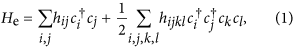where the operators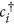and ci stand for the electrons and obey the fermionic anticommutation relations. Coefficients hij come from the single-electron integrals of the electron kinetic terms and electron-nuclei interactions and hijkl correspond to the two-electron integrals associated with the electron-electron Coulomb interaction. That is, it is expressed in atomic units as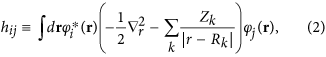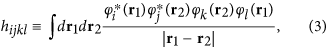where Rk are nuclear coordinates, r electronic coordinates and Z the atomic number representing the nuclear charge. Here, {φi(r)} defines a set of spin orbitals and r = (r, σ) denotes the pair of spatial and spin parameters.

Optimal strategies of computation for quantum chemistry merge quantum simulation and classical numerical techniques. These methods, that we name as algorithmic quantum simulation45, allow us to employ quantum simulators for the computationally hard tasks, such as time evolution, on top of the classical algorithm, which provides flexibility for computing relevant observables. In the context of quantum chemistry, we have the example of ground state finding via a variational eigensolver11,14,46,47,48.

The simulation of the dynamics associated with the electronic Hamiltonian in equation (1) involves fermionic operators. Computations with fermionic degrees of freedom in superconducting circuits require the encoding of fermionic operators and their anticommutative algebra in the natural variables of this quantum platform. The Jordan-Wigner transformation49 maps the fermionic operators into spin-1/2 operators, which gives us the qubit representation of the Hamiltonian. In the case of a hydrogen molecule, considering four electronic orbitals, the relations can be written as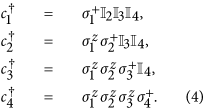After this mapping, the Hamiltonian of equation (1) for the H2 molecule is rewritten in terms of spin-1/2 operators considering only the nonzero coefficients hij and hijkl, which are computed classically with polynomial resources18,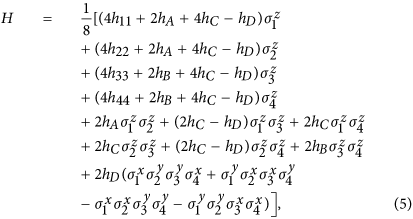where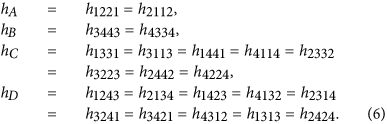In general, an analog quantum simulation of an arbitrary Hamiltonian evolution is a difficult problem50,51, since one cannot Straightforwardly map the dynamics of a given simulated system onto a given quantum platform. The flexibility and universality of digital quantum simulations allows us to reproduce models that do not appear naturally in a quantum platform. This is done via an expansion of the quantum evolution into discrete steps of quantum gates52. An additional advantage of such digital quantum simulations, in the spirit of gate-based quantum algorithms, is their possible improvement with quantum error correction techniques53,54.

We consider the digital quantum simulation of the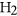molecule via the Trotter expansion, which consists in dividing the evolution time t into l time intervals of length t/l and applying sequentially the evolution operator of each term of the Hamiltonian for each time interval8,33,52. The expression of this expansion for a Hamiltonian of the form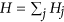reads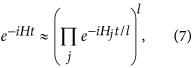for large l, where the dominating error component is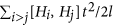, which depends on the value of the commutators and scales with t2/l.

In our case, we consider the evolution operators associated with the different summands of the Hamiltonian in equation (5), which corresponds to the sequence of gates in Fig. 1. We propose an algorithm based on the optimised tunable CZϕ gate, which allows one to perform efficiently ZZ interactions, or XX interactions in our basis28. In this sense, we arrange the gates and the simulated interactions such that it allows us to simplify the algorithm by eliminating some entangling gates and their inverses, as shown in Fig. 1. The single Trotter step depicted in this figure represents the approximated evolution for a time t/l of the complete Hamiltonian. Note that the third and fourth logical qubits correspond to the fourth and third physical qubits, respectively. We choose this notation due to the reduction of SWAP gates needed for the performance of the protocol. The optimized Trotter step contains 24 XX two-qubit gates between nearest-neighbour qubits, 24 SWAP gates and 20 single-qubit rotations. In Fig. 2, we show the efficiency of the digital protocol for different number of Trotter steps. Here, we analyze the loss of the state fidelity and the expected value of some operators performed in the simulation, considering simulated phases up to θ = h11t = 2. We break down the Hamiltonian terms and plot the energies of each of them to observe separately the Trotter error associated with the different kinds of interactions appearing in the algorithm. We observe that, for a single Trotter step, the energies related to single-qubit gates are similar to the exact evolution, while in the case of the four body terms the deviation is higher.

Symmetric Trotter expansions provide the improvement of the digital error at the expense of more gate execution. The Hamiltonian of equation (5) can be divided in two groups of interactions, H1, the sum of the first 10 terms that commute among them and H2, the sum of the last 4 terms that also commute among them. As commuting interactions do not generate digital error, the evolution of a symmetric Trotter step can be written as follows52,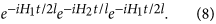This protocol requires the implementation of the interactions of H1 one more time than in the regular digital protocol, thereby reducing the digital error. We introduce a fixed error for any two-qubit gate between nearest-neighbour qubits, without restricting ourselves to a specific setup or experimental source of error. Single-qubit gate errors are neglected due to their high fidelity with current technology. If the dominating error is the experimental one, then the aim is to reduce the number of gates and, consequently, the regular protocol gets better fidelities. In Fig. 3, we analyze the errors of both the regular Trotter protocol and the symmetric protocol and we give an upper bound of the total error summing the digital and the experimental error considering a range of values for the two-qubit gate error employed. For fixed number of Trotter steps, l = 2, 3, 4, we observe crossing points between the errors associated with the symmetric protocol and the regular one whilst considering higher experimental gate error. On the left side of the crossing points, the experimental error is smaller and the symmetric protocol provides better results, whereas on the right side, as the experimental gate error grows, the regular protocol is more adequate. We also notice that, as the number of Trotter steps increases, the advantages of one protocol with respect to the other lessen. It is worthy to mention that the two-qubit gate errors are on the order of 10−2  in superconducting devices54.

Simulation of environmental effects

In this section, we propose a quantum simulation in superconducting circuits of generic system-environment interactions, which have long been recognised as fundamental in the description of electron transport in biomolecules.

Biological systems are not isolated and one can consider minimal models for characterising the quantum baths and decoherence25,26, such as the spin-boson model, or the Caldeira-Leggett model. The former is a widely used model that describes the interaction between a two-level system and a bosonic bath and the latter deals with the dynamics of a quantum particle coupled to a bosonic bath. Usually, the coupling of the quantum system to the bath degrees of freedom is completely specified by the spectral density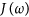, which may be obtained from experimental data and allows us to explore different continuum models of the environment. Nevertheless, in certain limits of strong coupling, the evaluations are computationally hard and the complete comprehension of the physics remains as an open problem.

In particular, we study a Hamiltonian describing the charge transfer in DNA wires42,43,44, where experiments show a wide range of results, from insulator to conductor behaviours55,56,57,58,59,60,61. When describing the dynamics of electrons in these biomolecules, the influence of a dissipative medium determines substantially the transfer events. We consider a bosonic bath in which a variety of crucial factors are contained, such as the internal vibrations of the biomolecule and the environmental effects.

A generic effective charge-bath model that describes an electronic system coupled to a fluctuating environment, in this case a bosonic bath, is captured by the Hamiltonian44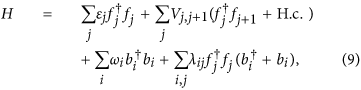with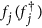, fermionic annihilation (creation) operators for electrons on different sites with energies εj. Vj,j+1 characterises the electron hopping between nearest-neighbour sites. The bath is represented by the bosonic annihilation (creation) operators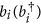and the coefficients λij indicate how the system and bath are coupled.

A minimal and particular case is the two-site model with j = A, B, which comprises a donor (D) and an acceptor (A) site42,43. This reduced model can be mapped onto the spin-boson model, which has been studied in cQED62, for the particular case of one spinless electron in the system. We provide the patterns to treat in a cQED setup a more general situation where the spin degree-of-freedom or more electrons enter into the description. To this end, we consider equation (9) with j = 1, 2, 3 and Vj,j+1 = V, which cannot be mapped onto the well-studied spin-boson model. For the sake of simplicity, we have chosen this truncation, but the techniques can be easily extrapolated to an arbitrary case.

As previously shown, in order to simulate fermionic operators in superconducting circuits, we replace them by Pauli matrices via the Jordan-Wigner transformation, leading to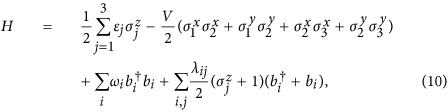where the first two terms correspond to the purely electronic subsystem, the third term is the free energy of the bosons in the bath and the last term represents the interaction of the electrons with the environment.

The Hamiltonian is now suitable for a digital quantum simulation in superconducting circuits, in which the qubits are described by Pauli operators and 3D cavities, multimode coplanar waveguides, or low-Q cavities play the role of bosonic baths. A first step in this direction, considering an open transmission line coupled to qubits in order to simulate fermionic systems interacting with a continuum of bosons was introduced in the context of quantum field theory35. While the basic protocol was already developed in this article, here we apply this formalism to the different context of electron transport in biomolecules, for a discrete set of coupled fermionic and bosonic modes. Recently, experimental realisations with a transmon qubit coupled to a multimode cavity in the strong coupling regime have been performed40. There, the feasibility of coupling a superconducting transmon qubit to a long coplanar resonator has been shown, achieving in this way the coupling of a qubit to a set of several bosonic modes at the same time. This multimode treatment is also needed to explain results in superconducting 3D cavities or in transmission lines39,41, which allows us to propose a simulation exploiting the natural complexity that superconducting circuits reveal.

By coupling three tunable superconducting qubits63,64 to a multimode cavity as in Fig. 4, the Hamiltonian of equation (10) can be reproduced by using digital-analog methods, that is, introducing the fermionic interactions digitally and the bosonic ones in analog interaction blocks. We propose the emulation of a variety of system-environment dynamics on superconducting circuit technology. To this end, we consider the interaction term describing theth qubit coupled to a multimode cavity,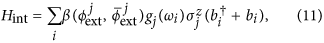withthe ith mode annihilation (creation) operator related with the cavity frequency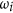, couplings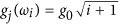and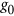the coupling strength to the fundamental cavity mode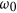. We profit from the tunability of the coupling between qubits and transmission lines via external magnetic fluxes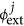and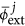35,63,64 to address a wider range of regimes and models, since the set of couplings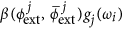mimic the coefficients λij that characterise the interaction with the environment in equation (10). Moreover, it has been shown experimentally how to engineer different shapes for the bath spectral function with a transmission line and partial reflectors62,65. Additionally, it can also be proven that a simple tunable Ohmic bath, as the one provided by a transmission line, equipped with a feedback protocol, can produce highly non-Markovian dynamics66. Growing in electronic complexity in equation (9) implies adding more qubits coupled to the transmission line in Fig. 4. However, we can take full advantage of the same multimode cavity by encoding the bath in a similar fashion. Hence, the cQED setup may be easily scaled up by coupling more qubits to the same transmission line.

Let us discuss how the Hamiltonian in equation (10) is decomposed into different digital and digital-analog blocks for the quantum simulation. As in the previous subsection, the purely electronic subsystem can be decomposed in Trotter steps and reproduced by single- and two-qubit gates. Since the bosonic operators do not enter in this part, we must decouple the tunable qubits from the transmission line to perform the required gates. The remaining terms are encoded in digital-analog blocks, where we divide the dynamics in different Trotter steps in which the multimode cavity enters in an analog way, providing the free energy of the bosons and simulating the last term of equation (10). This last term is composed of purely bosonic interactions proportional to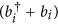, which may be simulated through a microwave driving in the cavity. It also involves qubit-boson interactions,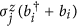, which emerge from the coupling of each qubit with the multimode cavity, as in ref. 35. A future analysis of the error in this protocol may include not only the error of the two-qubit gates, but also the contribution of the imperfect decoupling of the qubits with the transmission line in the single-qubit gates in which we introduce the coupling with the bosonic degrees of freedom. The figure of merit in the simulation is the final state of the fermions, which may be extracted from the final state of the qubits. The quantum simulation may be performed for different final times, thereby allowing us to reconstruct the electronic dynamics, such as transfer of excitations.

With this proposal, we have provided not only a way of extracting results illustrating different charge transport regimes in biomolecules, but also a way of testing different minimal models for describing molecules embedded in a bosonic environment. Superconducting circuits are a controllable quantum platform in which we can tune couplings between spins and bosons and manipulate external conditions to engineer different baths. We analyse models of biological systems with a certain complexity and translate them to a controllable superconducting device that enjoys a similar complexity.

Discussion

We have proposed methods to perform feasible digital and digital-analog quantum simulations of molecular structures and biomolecules with the state-of-the-art of superconducting circuit technology. We analyse different quantum chemistry models by increasing gradually the complexity, moving from purely fermionic models of molecular structures to descriptions of charge transport in biomolecules embedded in a bosonic medium. We aim to profit from the unique features of cQED, such as the strong coupling of a two-level system to bosonic modes, in order to represent controllable scenarios in which quantum chemistry and quantum biology models may be studied. The proposal includes a purely digital quantum simulation protocol for fermionic models, for which we provide general methods of encoding and the sequence of gates needed for the particular case of simulation of the H2 molecule. The previous formalism is partially used for simulating biomolecules affected by their bosonic surroundings, where we also add analog blocks with a multimode cavity playing the role of the bosonic bath, hence boosting the efficiency of quantum algorithms for quantum chemistry.

How to cite this article: García-Álvarez, L. et al. Quantum chemistry and charge transport in biomolecules with superconducting circuits. Sci. Rep. 6, 27836; doi: 10.1038/srep27836 (2016).

References

1. Szabo, A. & Ostlund, N. Modern Quantum Chemistry: Introduction to Advanced Electronic Structure Theory (Dover Publications, Mineola, NY 1996).

2. Helgaker, T., Jorgensen, P. & Olsen, J. Molecular Electronic-Structure Theory (Wiley, Chichester, UK 2000).

3. Sherrill, C. Frontiers in electronic structure theory. J. Chem. Phys. 132, 110902 (2010).

4. Whitfield, J. D., Love, P. J. & Aspuru-Guzik A. Computational complexity in electronic structure. Phys. Chem. Chem. Phys. 15, 397–411 (2013).

5. Aspuru-Guzik, A., Dutoi, A. D., Love, P. J. & Head-Gordon, M. Simulated quantum computation of molecular energies. Science 309, 1704–1707 (2005).

6. Kassal I., Whitfield, J. D., Perdomo-Ortiz, A., Yung, M.-H. & Aspuru-Guzik, A. Simulating chemistry using quantum computers. Annu. Rev. Phys. Chem. 62, 185–207 (2011).

7. Feynman, R. P. Simulating physics with computers. Int. J. Theor. Phys. 21, 467–488 (1982).

8. Lloyd, S. Universal quantum simulators. Science 273, 1073–1078 (1996).

9. Du, J. et al. NMR implementation of a molecular hydrogen quantum simulation with adiabatic state preparation. Phys. Rev. Lett. 104, 030502 (2010).

10. Lamata, L., Mezzacapo, A., Casanova, J. & Solano, E. Efficient quantum simulation of fermionic and bosonic models in trapped ions. EPJ Quantum Technology 1, 9 (2014).

11. Yung, M.-H. et al. From transistor to trapped-ion computers for quantum chemistry. Sci. Rep. 4, 3589 (2014).

12. Shen, Y. et al. Quantum implementation of unitary coupled cluster for simulating molecular electronic structure. Preprint at arXiv: 1506.00443 (2015).

13. Lanyon, B. P. et al. Towards quantum chemistry on a quantum computer. Nature Chem. 2, 106–111 (2010).

14. Peruzzo, A. et al. A variational eigenvalue solver on a photonic quantum processor. Nat. Commun. 5, 4213 (2014).

15. Huh, J., Guerreschi, G. G., Peropadre, B., McClean, J. R. & Aspuru-Guzik, A. Boson sampling for molecular vibronic spectra. Nature Photon. 9, 615–620 (2015).

16. Mostame, S. et al. Quantum simulator of an open quantum system using superconducting qubits: exciton transport in photosynthetic complexes. New J. Phys. 14, 105013 (2012).

17. Kassal, I., Jordan, S. P., Love, P. J., Mohseni, M. & Aspuru-Guzik, A. Polynomial-time quantum algorithm for the simulation of chemical dynamics. PNAS 105, 18681 (2008).

18. Whitfield, J. D., Biamonte, J. & Aspuru-Guzik, A. Simulation of electronic structure Hamiltonians using quantum computers. Mol. Phys. 109, 735–750 (2011).

19. Babbush, R., McClean, J., Wecker, D., Aspuru-Guzik, A. & Wiebe, N. Chemical basis of Trotter-Suzuki errors in quantum chemistry simulation. Phys. Rev. A 91, 022311 (2015).

20. Babbush, R., Love, P. J. & Aspuru-Guzik, A. Adiabatic quantum simulation of quantum chemistry. Sci. Rep. 4, 6603 (2014).

21. Poulin, D. et al. The Trotter step size required for accurate quantum simulation of quantum chemistry. Quantum Inf. Comput. 15, 361–384 (2015).

22. Whitfield, J. D. Unified views of quantum simulation algorithms for chemistry. Preprint at arXiv: 1502.03771 (2015).

23. Hastings, M. B., Wecker, D., Bauer, B. & Troyer, M. Improving quantum algorithms for quantum chemistry. Quantum Inf. Comput. 15, 1–21 (2015).

24. Toloui, B. & Love, P. J. Quantum algorithms for quantum chemistry based on the sparsity of the CI-matrix. Preprint at arXiv: 1312.2579 (2013).

25. Abbott, D., Davies, P. C. W. & Pati, A. K. Quantum Aspects of Life (Imperial College Press 2008).

26. May, V. & Kühn, O. Charge and Energy Transfer Dynamics in Molecular Systems (WILEY-VCH Verlag GmbH & Co. KGaA, Weinheim 2011).

27. Devoret, M. H. & Schoelkopf, R. J. Superconducting circuits for quantum information: an outlook. Science 339, 1169–1174 (2013).

28. Barends, R. et al. Digital quantum simulation of fermionic models with a superconducting circuit. Nat. Commun. 6, 7654 (2015).

29. Salathé, Y. et al. Digital quantum simulation of spin models with circuit quantum electrodynamics. Phys. Rev. X 5, 021027 (2015).

30. Barends, R. et al. Digitized adiabatic quantum computing with a superconducting circuit. Preprint at arXiv: 1511.03316 (2015).

31. Las Heras, U. et al. Digital quantum simulation of spin systems in superconducting circuits. Phys. Rev. Lett. 112, 200501 (2014).

32. Mezzacapo, A. et al. Digital quantum Rabi and Dicke models in superconducting circuits. Sci. Rep. 4, 7482 (2014).

33. Las Heras, U., García-Álvarez, L., Mezzacapo, A., Solano, E. & Lamata, L. Fermionic models with superconducting circuits. EPJ Quantum Technology 2, 8 (2015).

34. Chiesa, A. et al. Digital quantum simulators in a scalable architecture of hybrid spin-photon qubits. Sci. Rep. 5, 16036 (2015).

35. García-Álvarez, L. et al. Fermion-fermion scattering in quantum field theory with superconducting circuits. Phys. Rev. Lett. 114, 070502 (2015).

36. O’Malley, P. J. J. et al. Scalable quantum simulation of molecular energies. Preprint at arXiv: 1512.06860 (2015).

37. Egger, D. J. & Wilhelm, F. K. Multimode circuit quantum electrodynamics with hybrid metamaterial transmission lines. Phys. Rev. Lett. 111, 163601 (2013).

38. Krimer, D. O., Liertzer, M., Rotter, S. & Türeci, H. E. Route from spontaneous decay to complex multimode dynamics in cavity QED. Phys. Rev. A 89, 033820 (2014).

39. Nigg, S. E. et al. Black-box superconducting circuit quantization. Phys. Rev. Lett. 108, 240502 (2012).

40. Sundaresan, N. M. et al. Beyond strong coupling in a multimode cavity. Phys. Rev. X 5, 021035 (2015).

41. McKay, D. C., Naik, R., Reinhold, P., Bishop, L. S. & Schuster, D. I. High-contrast qubit interactions using multimode cavity QED. Phys. Rev. Lett. 114, 080501 (2015).

42. Bulla, R., Gutiérrez, R. & Cuniberti, G. Modelling molecular conduction in DNA wires: charge transfer theories and dissipative quantum transport In Modern Methods for Theoretical Physics Chemistry of Biopolymers (Elsevier, Amsterdam 2006).

43. Tornow, S., Tong, N.-H. & Bulla, R. Electron transfer in donor-acceptor systems: many-particle effects and influence of electronic correlations. Europhys. Lett. 73, 913 (2006).

44. Gutiérrez, R. et al. Charge transport through biomolecular wires in a solvent: bridging molecular dynamics and model Hamiltonian approaches. Phys. Rev. Lett. 102, 208102 (2009).

45. Alvarez-Rodriguez, U., Di Candia, R., Casanova, J., Sanz, M. & Solano, E. Algorithmic quantum simulation of memory effects. Preprint at arXiv: 1604.02992 (2016).

46. Wecker, D., Hastings, M. B. & Troyer, M. Progress towards practical quantum variational algorithms. Phys. Rev. A 92, 042303 (2015).

47. Bauer, B., Wecker, D., Millis, A. J., Hastings, M. B. & Troyer, M. Hybrid quantum-classical approach to correlated materials. Preprint at arXiv:1510.03859 (2015).

48. McClean, J. R., Romero, J., Babbush, R. & Aspuru-Guzik, A. The theory of variational hybrid quantum-classical algorithms. New J. Phys. 18, 023023 (2016).

49. Jordan, P. & Wigner, E. Über das Paulische Äquivalenzverbot. Z. Phys. 47, 631 (1928).

50. Mei, F., Stojanović, V. M., Siddiqi, I. & Tian, L. Analog superconducting quantum simulator for Holstein polarons. Phys. Rev. B 88, 224502 (2013).

51. Seo, K. & Tian, L. Quantum phase transition in a multiconnected superconducting Jaynes-Cummings lattice. Phys. Rev. B 91, 195439 (2015).

52. Suzuki, M. Fractal decomposition of exponential operators with applications to many-body theories and Monte Carlo simulations. Phys. Lett. A 146, 319–323 (1990).

53. Trout, C. J. & Brown, K. R. Magic state distillation and gate compilation in quantum algorithms for quantum chemistry. Int. J. Quantum Chem. 115, 1296–1304 (2015).

54. Barends, R. et al. Logic gates at the surface code threshold: superconducting qubits poised for fault-tolerant quantum computing. Nature 508, 500–503 (2014).

55. Porath, D., Bezryadin, A., de Vries, S. & Dekker, C. Direct measurement of electrical transport through DNA molecules. Nature 403, 635–638 (2000).

56. Storm, A. J., Noort, J. V., de Vries, S. & Dekker, C. Insulating behavior for DNA molecules between nanoelectrodes at the 100 nm length scale. Appl. Phys. Lett. 79, 3881 (2001).

57. Yoo, K.-H. et al. Electrical Conduction through Poly(dA)-Poly(dT) and Poly(dG)-Poly(dC) DNA Molecules. Phys. Rev. Lett. 87, 198102 (2001).

58. Long-Range Charge Transfer in DNA I and II. Topics in Current Chemistry Vol. 237 (eds Schuster, G. B. ) (Springer, Berlin 2004).

59. Xu, B., Zhang, P., Li, X. & Tao, N. Direct conductance measurement of single DNA molecules in aqueous solution. Nano Lett. 4, 1105–1108 (2004).

60. Cohen, H., Nogues, C., Naaman, R. & Porath, D. Direct measurement of electrical transport through single DNA molecules of complex sequence. Proc. Natl. Acad. Sci. USA 102, 11589–93 (2005).

61. Nogues, C., Cohen, S. R., Daube, S., Apter, N. & Naaman, R. Sequence dependence of charge transport properties of DNA. J. Phys. Chem. B 110, 8910–8913 (2006).

62. Haeberlein, M. et al. Spin-boson model with an engineered reservoir in circuit quantum electrodynamics. Preprint at arXiv: 1506.09114 (2015).

63. Srinivasan, S. J., Hoffman, A. J., Gambetta, J. M. & Houck, A. A. Tunable coupling in circuit quantum electrodynamics using a superconducting charge qubit with a v-shaped energy level diagram. Phys. Rev. Lett. 106, 083601 (2011).

64. Chen, Y. et al. Qubit architecture with high coherence and fast tunable coupling. Phys. Rev. Lett. 113, 220502 (2014).

65. Forn-Díaz, P. et al. Ultrastrong coupling of a single artificial atom to an electromagnetic continuum. Preprint at arXiv: 1602.00416 (2016).

66. Pfeiffer, P., Egusquiza, I. L., Di Ventra, M., Sanz, M. & Solano, E. Quantum memristors. Preprint at arXiv: 1511.02192 (2015).

Acknowledgements

We acknowledge support from two UPV/EHU PhD grants, UPV/EHU Project EHUA14/04, Basque Government IT472-10; Spanish MINECO FIS2012-36673-C03-02 and FIS2015-69983-P; Ramón y Cajal Grant RYC-2012-11391; and SCALEQIT EU projects.

Author information

Authors

Contributions

L.G.-Á. and U.L.H. designed the protocol in Figure 1, did the calculations and numerical analysis and prepared the figures. L.G.-Á., U.L.H., A.M., M.S., E.S. and L.L. contributed developing ideas, analysing results and writing the manuscript.

Ethics declarations

Competing interests

The authors declare no competing financial interests.

Rights and permissions

Reprints and Permissions

• Digital-analog quantum simulation of generalized Dicke models with superconducting circuits

• Lucas Lamata

Scientific Reports (2017)